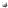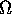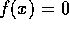Back to the Home-Page

# Steve Smale1951-601961-701971-801981-901991-20001. A note on open maps, Proceedings of the AMS, 8 (1957), pp. 391--393.

2. A Vietoris mapping theorem for homotopy, Proceedings of the AMS, 8 (1957), pp. 604--610.

3. Regular curves on Riemannian manifolds, Transactions of the AMS, 87 (1958), pp. 492--512.

4. On the immersions of manifolds in Euclidean space (with R. K. Lashof), Annals of Mathematics, 68 (1958), pp. 562--583.

5. Self-intersections of immersed manifolds (with R. K. Lashof), Journal of Mathematics and Mechanics, 8 (1959), pp. 143--157.

6. A classification of immersions of the two-sphere, Transactions of the AMS, 90 (1959), pp. 281--290.

7. The classification of immersions of spheres in Euclidean space, Annals of Mathematics, 69 (1959), pp. 327--344. (See also R. Thom, La Classification des immersions d'apres Smale, Seminaire Bourbaki, December 1957.)

8. Diffeomorphisms of the two-sphere, Proceedings of the AMS, 10 (1959), pp. 621--626.

9. On involutions of the 3-sphere (with Morris Hirsch), American Journal of Mathematics, 81 (1959), pp. 893--900.

10. Morse inequalities for a dynamical system, Bulletin of the AMS, 66 (1960), pp. 43--49.

11. The generalized Poincaré conjecture in higher dimensions, Bulletin of the AMS, 66 (1960), pp. 373--375.

12. On dynamical systems, Boletin de la Sociedad Mathematics Mexicana, (1960), pp. 195--198.

13. On gradient dynamical systems, Annals of Mathematics, 74 (1961), pp. 199--206.

14. Generalized Poincaré conjecture in dimensions greater than 4, Annals of Mathematics, 74 (1961), pp. 391--406.

15. Differentiable and combinatorial structures on manifolds, Annals of Mathematics, 74 (1961), pp. 498--502.

16. On the structure of 5-manifolds, Annals of Mathematics, 75 (1962), pp. 38--46.

17. On the structure of manifolds, American Journal of Mathematics, 84 (1962), pp. 387--399.

18. Dynamical systems and the topological conjugacy problem for diffeomorphisms, Proceedings of the International Congress of Mathematicians, Stockholm, 1962.

19. A survey of some recent developments in differential topology, Bulletin of the AMS, 69 (1963), pp. 131--146.

20. Stable manifolds of diffeomorphisms and differential equations, Annali della Scuola Normali Superioro di Pisa, Serie III, XVII (1963), pp. 97--116.

21. A generalized Morse theory (with R. Palais), Bulletin of the AMS, 70 (1964), pp. 165--172.

22. Morse theory and non-linear generalization of the Dirichlet problem, Annals of Mathematics, 80, pp. 382--396.

23. Diffeomorphisms with many periodic points, Differential and Combinatorial Topology (A symposium in honor of Marston Morse), Princeton University Press (1965), pp. 63--80.

24. An infinite dimensional version of Sard's theorem, American Journal of Mathematics, 87 (1965), pp. 861--866.

25. On the Morse index theorem, Journal of Mathematics and Mechanics, 14 (1965), pp. 1049--1056.

26. A structurally stable differentiable homeomorphism with an infinite number of periodic points, Report on the Symposium on Non Linear Oscillations, Kiev Mathematics Institute (1963), pp. 365--366.

27. On the calculus of variations, Differential Analysis, Bombay (1964), pp. 187--189.

28. Structurally stable systems are not dense, American Journal of Mathematics, 88 (1966), pp. 491--496.

29. Dynamical systems on n-dimensional manifolds, Differential Equations and Dynamical Systems, (1967), pp. 483--486.

30. Differentiable dynamical systems, Bulletin of the AMS, 73 (1967), pp. 747--817.

31. What is global analysis?, American Math. Monthly, (1969), pp. 4--9.

32. Nongenericity of-stability (with R. Abraham), Global Analysis, Proc. of Symposia in Pure Mathematics, 14 AMS (1970), pp. 5--8.

33. Structural stability theorems (with J. Palis), Global Analysis, Proc. of Symposia in Pure Mathematics, 14 AMS (1970), pp. 223--231.

34. Notes on differential dynamical systems, Global Analysis, Proc. of Symposia in Pure Mathematics, 14 AMS (1970), pp. 277--287.

35. The-stability theorem, Global Analysis, Proc. of Symposia in Pure Mathematics, 14 AMS (1970), pp. 289--297.

36. Topology and mechanics, I., Inventiones Mathematicae, 10 (1970), pp. 305--331.

37. Topology and mechanics, II., Inventiones Mathematicae, 11 (1970), pp. 45--64.

38. Stability and genericity in dynamical systems, Seminaire Bourbaki, (1969--70), Springer, Berlin, pp. 177--186.

39. Problems on the nature of relative equilibria in celestial mechanics, Proc. of Conference on Manifolds, Amsterdam (1970), Springer, Berlin, pp. 194--198.

40. On the mathematical foundations of electrical circuit theory, Journal of Differential Geometry, 7 (1972), pp. 193--210.

41. Beyond hyperbolicity (with M. Shub), Annals of Mathematics, 96 (1972), pp. 591--597.

42. Personal perspectives on mathematics and mechanics, Statistical Mechanics: New Concepts, New Problems, New Applications, edited by Stuart A. Rice, Karl F. Freed, and John C. Light, Univ. of Chicago Press (1972), pp. 3--12.

43. Stability and isotopy in discrete dynamical systems, Dynamical Systems, edited by M. M. Peixoto, Academic Press, New York (1973).

44. Global analysis and economics, I: Pareto Optimum and a generalization of Morse theory, Dynamical Systems, edited by M. M. Peixoto, Academic Press (1973).

45. Global analysis and economics, IIA, Journal of Mathematical Economics, First Issue, April 1974, pp. 1--14.

46. Global analysis and economics, III: Pareto Optima and price equilibria, Journal of Mathematical Economics, I (1974), pp. 107--117.

47. Global analysis and economics, IV: Finiteness and stability of equilibria with general consumption sets and production, Journal of Mathematical Economics, I (1974), pp. 119--127.

48. Global analysis and economics, V: Pareto theory with constraints, Journal of Mathematical Economics, I (1974), pp. 213--221.

49. Optimizing several functions, Manifolds, Tokyo (1973), Proc. of the International Conference on Manifolds and Related Topics in Topology, Tokyo (1973), Univ. of Tokyo Press (1975), pp. 69--75.

50. The mathematical model of two cells via Turing's equation, Lectures on Mathematics in the Life Sciences, 6 (1974), pp. 17--26.

51. Sufficient conditions for an optimum, Warwick Dynamical Systems (1974), Lecture Notes in Mathematics, Springer--Verlag (1975), pp. 287--292.

52. Differential equations (with I. M. Singer), Encyclopedia Britannica Inc., Fifteenth Edition, (1974), pp. 736--767.

53. Dynamics in general equilibrium the theory, American Economic Review, 66 (1976), pp. 288--294.

54. Global analysis and economics, VI: Geometric analysis of Parieto Optima and price equilibria under classical hypotheses, Journal of Mathematical Economics, 3 (1976), pp. 1--14.

55. The qualitative analysis of a difference equation of population growth (with R. F. Williams), Journal of Mathematical Biology, 3 (1976), PP. 1--4.

56. On the differential equations of species in competition, Journal of Mathematical Biology, 3 (1976), pp. 5--7.

57. A convergent process of price adjustment, Journal of Mathematical Economics, 3 (1976), pp. 107--120.

58. Exchange processes with price adjustment, Journal of Mathematical Economics, 3 (1976), pp. 211--226.

59. Global stability questions in dynamical systems, Proceedings of Conference on Qualitative Analysis, Washington, DC.

60. Dynamical systems and turbulence, Turbulence Seminar: Berkeley 1976/77, edited by P. Bernard and T. Ratiu, Lecture Notes in Mathematics, 615 (1977), pp. 48--71.

61. Some dynamical questions in mathematical economics, Colloques Internationaux du Centre National de la Recherche Scientifique, No. 259: Systemes Dynamiques et Modeles Economiques, (1977).

62. An approach to the analysis of dynamic processes in economic systems, Equilibrium and disequilibrium in Economic Theory, D. Reidel Publishing Co., Dordrecht, (1977), pp. 363--367.

63. On comparative statics and bifurcation in economic equilibrium theory, Annals of the New York Academy of Sciences, 316 (1979), (Bifurcation Theory in Scientific Disciplines), pp. 545--548.

64. On algorithms for solving(with Morris W. Hirsch), Communications on Pure and Applied Mathematics, 55 (1980), pp. 1--12.

65. Smooth solutions of the heat and wave equations, Commentarii Mathematici Helvetici, 55 (1980), pp. 1--12.

66. The fundamental theorem of algebra and complexity theory, Bulletin of the American Mathematical Society, 4 (1981), pp. 1--36.

67. The prisoner's dilemma and dynamical systems associated to non-cooperative games, Econometrica, 48 (1980), pp. 1617--1634.

68. Global analysis and economics, Handbook of Mathematical Economics, K. J. Arrow and M. D. Intrilligator, North-Holland, Amsterdam, 1 (1981), pp. 331--370.

69. The Mathematics of Time: Essays on Dynamical Systems, Economic Processes and Related Topics, Springer--Verlag, Berlin, (1980), vi + pp. 151.

70. The problem of the average speed of the simplex method, Proceedings of the XIth International Symposium on Mathematical Programming, edited by Bachem, Grotschel, and Korte, Springer--Verlag, Berlin, (1983), pp. 530--539.

71. On the average number of steps of the simplex method of linear programming, Mathematical Programming, 27 (1983), pp. 241--262.

72. On the steps of Moscow University, Mathematical Intelligencer, 6 (1984), pp. 21--27.

73. Gerard Debreu wins the Nobel Prize, Mathematical Intelligencer, 6 (1984), pp. 61--62.

74. Scientists and the arms race (in German translation), Natur-Wissenschafter Gegen Atomrustung, edited by Hans-Peter Durr, Hans-Peter Harjes, Matthias Krech, and Peter Starlinge, Spiegel--Buch, (1983), pp. 327--334.

75. Computational complexity: On the geometry of polynomials and a theory of cost, Part I (with Mike Shub), Annales Scientifiques de l'Ecole Normale Superieure, 18 (1985), pp. 107--142.

76. On the efficiency of algorithms of analysis, Bulletin of the American Mathematical Society, 13 (1985), pp. 87--121.

77. Computational complexity: On the geometry of polynomials and a theory of cost, Part II (with Mike Shub), SIAM Journal of Computing, 15 (1986), pp. 145--161.

78. On the existence of generally convergent algorithms (with Mike Shub), Journal of Complexity, 2 (1986), pp. 2--11.

79. Newton's method estimates from data at one pint, The Merging of Disciplines: New Directions in Pure, Applied, and Computational Mathematics, edited by Richard E. Ewing, Kenneth I. Gross, and Clyde F. Martin, Springer--Verlag, New York, (1986), pp. 185--196.

80. On the topology of algorithms, I., Journal of Complexity, 3 (1987), pp. 81--89.

81. Algorithms for solving equations, Proceedings of the International Congress of Mathematicians, August 3--11, 1986, Berkeley, California, USA, American Mathematical Society Providence, (1987), pp. 172--195.

82. Global analysis in economic theory, The New Palgrave: A Dictionary of Economics, 2, edited by John Eatwell, Murray Milgrate, and Peter Newman, Macmillan Press, (1987), London, pp. 532--534.

83. The Newtonian contribution to our understanding of the computer, Queen's Quarterly, 95 (1988), pp. 90--95.

84. On a theory of computation and complexity over the real numbers: NP-completeness, recursive functions and universal machines (with Lenore Blum and Mike Shub), Bulletin (New Series) of the American Mathematical Society, 21(1) (1989), pp. 1--46.

85. Some remarks on the foundations of numerical analysis, SIAM Review, 32(2) (1990), pp. 221--270.

86. The story of the higher dimensional Poincaré conjecture, The Mathematical Intelligencer, 21(2) (1990), pp. 40--51.

87. Dynamics retrospective: great problems, attempts that failed, Physica D, 51 (1991), pp. 267--273.

88. Theory of computation, Mathematical Research Today Tomorrow, ed. Casacuberta, Castellet Spring, New York, (1992), pp. 60--69.

89. Complexity of Bezout's Theorem I: Geometric aspects (with Mike Shub), Journal of the American Mathematical Society, 6 (1993), pp. 459--501.

90. Complexity of Bezout's Theorem II: Volumes and probabilities (with Mike Shub), Computational Algebraic Geometry (F. Eyssette and A. Galligo, eds.) Progress in Mathematics, Birkhauser, 109 (1993), pp. 267--285.

91. Complexity of Bezout's Theorem III: Condition number and packing (with Mike Shub), Journal of Complexity, 9 (1993), pp. 4--14.

92. What is Chaos, in Nobel Conference XXVI, Chaos the New Science, ed. J. Holte Gustavus Adolphus College, University Press in American, (1993), pp. 89--104.

93. Complexity of Bezout's Theorem V: Polynomial Time (with Mike Shub), Theoretical Computer Science, 133 (1994), pp. 141--164

94. Separation of Complexity Classes in Koiran's Weak Model (with Felipé Cucker and Mike Shub), Theoretical Computer Science, 133 (1994), pp. 3--14.

95. Complexity of Bezout's Theorem IV: Probabability of success; Extensions (with Mike Shub), SIAM Jour. of Numerical Analysis (1996).

96. On the Intractibility of Hilbert's Nullstellensatz and an Algebraic Version of "NP=P?" (with Mike Shub) Duke Math Jour to appear.

97. Complexity and Real Computation: A Manifesto (with L. Blum, F. Cucker and M. Shub) International Journal of Bifurcation and Chaos Vol. 6 No.1 (1996).

98. Algebraic Settings for the Problem "P=NP" (with L. Blum, F. Cucker, M. Shub) Lectures in Applied Mathematics, ed J. Renegar, M. Shub and S. Smale to appear

99. Chaos: Finding a Horseshoe on the Beaches of Rio to appear

Back to the top

Steve Smale
Last update: April 1996